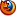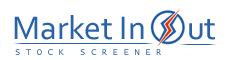Please enable JavaScript to view this page content properly Log In | Sign Up
 Pre-Tax Profit Margin Leverage Ratio Current Ratio Price / Book Ratio Price / Tangible Book Ratio Price / Cash Flow Ratio Price / Free Cash Flow Ratio Debt / Equity Ratio Price / Sales Ratio Income per Employee
 Debt / Equity Ratio Action Debt / Equity Ratio: More than 20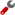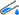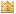Debt / Equity Ratio: 10 to 20Debt / Equity Ratio: 8 to 10Debt / Equity Ratio: 5 to 8Debt / Equity Ratio: 3 to 5Debt / Equity Ratio: 2 to 3Debt / Equity Ratio: 1 to 2Debt / Equity Ratio: 0 to 1Best viewed in